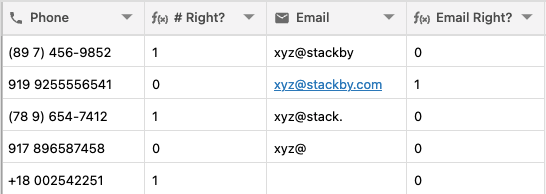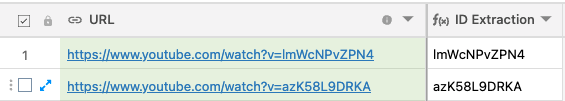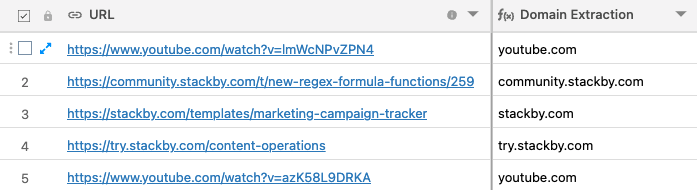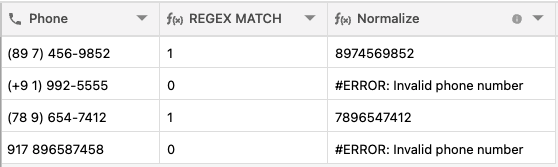# Guide to REGEX() functionsBy Anjali Daga

###### updated 2 months ago

Regular expressions allow you to find patterns in character combinations in a string. It's super powerful and are used in modern search engines and word processors.

There are three types of REGEX Formula functions in Stackby. They are REGEX_MATCH(), REGEX_EXTRACT(), and REGEX_REPLACE(). All this are formula which has advantages and disadvantages depending on the formula output.

Here you can learn how REGEX functions work.

In this article, we will learn the following Regular expression functions:

• REGEX_MATCH()
• REGEX_EXTRACT()
• REGEX_REPLACE()

## 1. REGEX_MATCH

The REGEX_MATCH() function returns whether the input text matches a regular expression and outputs in the Boolean data type as a 1 as true or 0 as false result.

Example: phone number validation and email validation.
Phone No.: REGEX_MATCH( {Phone}, ‘^([+]?[0-9]( |-)?)?(\\(?[0-9]{3}\\)?|[0-9]{3})( |-)?([0-9]{3}( |-)?[0-9]{4}|[a-zA-Z0-9]{7})\$‘)
Email: REGEX_MATCH({Email}, “(\\W|^)[\\w.\\-]{0,25}@[A-Za-z0-9.-]+\\.[A-Za-z]{2,}(\\W|\$)“)## 2. REGEX_EXTRACT()

In the REGEX_EXTRACT() function it returns the first substring that matches a regular expression. If the REGEX_EXTRACT() function finds no matching instance of the provided regular expression it will return an ERROR.

Example: Extracting ID from a URL
Formula: REGEX_EXTRACT({URL}, “[^=]+\$“)Another example: Domain Extraction
Formula: REGEX_EXTRACT( {URL}, ‘^(?:https?:\\/\\/)?(?:[^@\n]+@)?(?:www\\.)?([^:\\/\n?]+)’)## 3. REGEX_REPLACE()

The REGEX_REPLACE() function substitutes all matching substrings with a replacement string value.

Let’s see if we have to normalize all our phone numbers in the table, we will follow the steps below:

We’ll first check if we have a valid phone number with REGEX_MATCH, then we can use REGEX_REPLACE to bring an output for a normalized phone number.

Step 1: First use REGEX_MATCH: REGEX_MATCH( {Phone}, ‘^([+]?[0-9]( |-)?)?(\\(?[0-9]{3}\\)?|[0-9]{3})( |-)?([0-9]{3}( |-)?[0-9]{4}|[a-zA-Z0-9]{7})\$‘)

Step 2: Then use REGEX_REPLACE to normalize: IF({REGEX MATCH}, UPPER(REGEX_REPLACE( {Phone}, ‘[^A-Za-z0-9]‘, ‘’)), ERROR(‘Invalid phone number’))